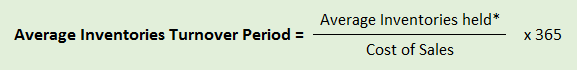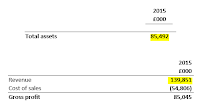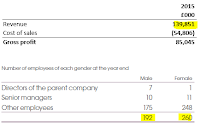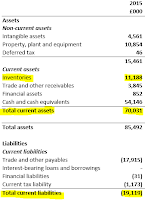## Pages

### 3 Efficiency and Liquidity Ratios

Financial ratios can be a relatively easy way to assess various aspects of a company's performance. Spanning profitability, liquidity, efficiency, stability and valuation, many of these ratios are very common, and when the results of these ratios are compared to other companies in the sector, or on the same company over time, they can be a simple yet powerful tool in uncovering the underlying progress of the company against peers. This tutorial will look at three efficiency ratios and three liquidity ratios; liquidity ratios look at the ability of a company to pay its short-term liabilities. This tutorial will cover those that are most easy to calculate given the information required is commonplace in financial statements.

Financial ratios are most useful when comparing like-for-like companies. For these ranges of efficiency and liquidity ratios we will look at two companies that are very similar in terms of operation: Boohoo (LSE:BOO) and ASOS (LSE:ASC). Both of these companies is involved in the production, distribution and sale of clothing solely through online websites, on a global scale. I will demonstrate how to calculate each of these ratios for Boohoo and then compare it with the figure for ASOS. We will use the 2014 results for Boohoo, which can be found here.

Ratios 1 to 3 cover efficiency ratios. Ratios 4 to 6 cover liquidity ratios.

1. Average Inventories Turnover Period (AITP)Take the inventories value for the year under scrutiny and add it to that of the previous year, and divide by 2
AITP, measured in days (always round up), tells an investor how long it takes to sell inventories after they have purchased. An increasing or long inventories turnover period could suggest difficulty with selling the inventories, or might suggest that the company's inventory control is inefficient. Bear in mind that this figure may be seasonally affected.Click to Enlarge
Boohoo Calculation: For this we need to identify the two of the equation. For average inventories held we need to identify the inventories figure for 2014 and the inventories figure for 2015 from the balance sheet. We then need to add the two together and divide by 2, to get the average inventories. The cost of sales can be found on the income statement. The relevant figures and parts of the financial statements references can be found in the image to the right.
Let's start by calculating the average inventories:﻿﻿﻿
Average Inventories = (11188+9795)/2 = 10491.5

We can now compute the ratio:
AITP = (10491.5/54806) * 365 = 70 days

This compares to ASOS's AITP of 114 days. You could conclude that, on this ratio, Boohoo appears to have more efficient inventory control.

2. Asset Turnover Ratio

Asset turnover, measured as a multiple, seeks to identify how much turnover is produced per unit of total assets held. A higher multiple implies that the assets are capable of generating higher revenues, hence a higher multiple is preferable. However, this is a highly sector specific ratio so only use when comparing two companies in the same sector.
﻿﻿Click to Enlarge
Boohoo Calculation: The revenue figure can be found at the top of the income statement with the total assets from the balance sheet. The relevant figures and parts of the financial statements references can be found in the image to the right.

We can now compute the ratio:
Asset Turnover = 139851/85492 = 1.64 times

This compares to ASOS's asset turnover of 2.57 times. ASOS appears to generate a higher level of revenue from its current asset base, implying that it is more efficient in this respect.

3. Revenue per Employee

Revenue per employee, measured in £s, is a direct measure of efficiency by looking at the average revenue made per employee; it's a crude measure of productivity. The higher the figure the better. This ratio is particularly useful in service sectors such as consultancy where employees are directly the revenue generators. Although you may choose to exclude directors from the number of employees, it's generally OK to include them if for simplicity.
﻿Click to Enlarge

Boohoo Calculation: We have already calculated the revenue figure from the asset turnover multiple, hence it remains to find the number of employees that Boohoo hires. This can often be found on the company's last annual report through a quick search (CTRL+F). The relevant figures can be found in the image to the right.

We can now compute the ratio:
Revenue per Employee = £139,851,000 / (192+260) = £309,405 per employee

This compares to ASOS's revenue per employee of £538,042. ASOS's figure is superior to that of Boohoo. However, given that these specific business models revolve around online sales, this is not the most meaningful read-through; the individual employees are not those who are generating the sales.

4. Current Ratio

The Current Ratio, measured as a multiple, is a direct method of telling whether a company's short-term assets are capable of covering the short-term liabilities. From a purely liquidity standpoint, the higher the multiple the better. A current ratio less than 1.0 could suggest the company will struggle to pay its short-term liabilities, hence can be perceived to be higher risk.
﻿Click to Enlarge
Boohoo Calculation: The two figures required are both located on the balance sheet and are relatively easy to find. The relevant figures and parts of the financial statements references can be found in the image to the right.

We can now compute the ratio:
Current Ratio = 70031/19119 = 3.66 times

This compares to ASOS's current ratio of 1.40. Both of these current ratios are far in excess of the 1.0 level pivotal level, suggesting that both companies are in a position whereby they should not struggle to meet their short-term liabilities using the short-term assets. Boohoo's current ratio is superior from a purely liquidity standpoint.

5. Acid Test Ratio

The acid test ratio, measured as a multiple, is a modification of the current ratio where we subtract inventories from current assets on the numerator. This is to generate a 'worst case scenario' where a company may be unable to sell its inventories in a timely matter, hence the acid test ratio will nearly always produce a lower figure compared to the current ratio. An acid-test ratio less than 0.7 could suggest the company is high risk (unless the business is required to hold high levels of inventories). The higher the calculated multiple, the better.
﻿Click to Enlarge
Boohoo Calculation: We already have the current assets and current liabilities figures from the current ratio, hence we simply have to find the inventories value from the balance sheet under the assets section. The relevant figures and parts of the financial statements references can be found in the image to the right.﻿

We can now compute the ratio:
Acid Test Ratio = 58843/19119 = 3.08 times

This compares to ASOS's acid test ratio of 0.53. We would expect this given that Boohoo's current ratio was higher than ASOS's. Although ASOS's acid test ratio is less than 0.7, their business model revolves around online sales of clothing hence they will always have a large stock of inventory. Therefore, you could waive the 0.7 rule of thumb in this instance. Nevertheless, Boohoo has a stronger acid test ratio than ASOS.

6. Operating Cash Flow Ratio

The operating cash flow ratio, measured as a multiple, tells an investor how many times the cash flow from operating activities covers the current liabilities of the business. The higher the multiple, the better, as it implies cash flow from operations can easily cover the current liabilities of the business, hence liquidity is perhaps not a serious issue.Click to Enlarge
Boohoo Calculation: We already have the current liabilities figure from previous calculations. To find the cash flow from operating activities figure, we have to look at the cash flow statement. The relevant figures and parts of the financial statements references can be found in the image to the right.

We can now compute the ratio:
Operating cash flow ratio = 12161/19119 = 0.64 times

This compares to ASOS's operating cash flow ratio of 0.37. Again, you would expect this since Boohoo has a much smaller liabilities base compared to ASOS. This says that ASOS could pay back 37% of its liabilities out of its cash flow from operating activities, whereas Boohoo could pay back 64% of its liabilities out of its cash flow from operating activities. Boohoo again appears to have superior liquidity.

Conclusion: Although Boohoo dominates the liquidity ratios, it only had superior efficiency within the AITP category.

Does that make Boohoo worth buying over ASOS? No. We would need to check the relative valuations of each, compare the two companies on profitability ratios and conduct other research into the outlook for each company.

This tutorial has looked at 3 efficiency and liquidity ratios that you can apply when conducting fundamental analysis, and can be applied to all cases.